International
Tables for
Crystallography
Volume F
Crystallography of biological macromolecules
Edited by E. Arnold, D. M. Himmel and M. G. Rossmann

International Tables for Crystallography (2012). Vol. F, ch. 11.4, pp. 289-291   | 1 | 2 |

Section 11.4.8. Scaling – multiplicative corrections

Z. Otwinowski,a* W. Minor,b D. Boreka and M. Cymborowskib

aUT Southwestern Medical Center at Dallas, 5323 Harry Hines Boulevard, Dallas, TX 75390–9038, USA, and bDepartment of Molecular Physiology and Biological Physics, University of Virginia, 1300 Jefferson Park Avenue, Charlottesville, VA 22908, USA
Correspondence e-mail:  zbyszek@work.swmed.edu

11.4.8. Scaling – multiplicative corrections

| top | pdf |

The relation between the measured intensity I(hkl) of reflection hkl and its squared structure-factor amplitude |F(hkl)|2 is described by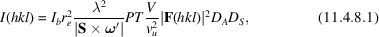where Ib is the flux density of the primary beam;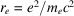is the classical electron radius (2.818 × 10−12 mm); λ is the wavelength of the beam;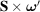is a cross product between the diffraction vector S and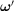(the projection of the crystal-rotation-speed vectoron the plane perpendicular to the primary beam; thus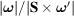is the Lorentz factor); P is a polarization factor (Azaroff, 1955); T is the transmission of the beam [related to the absorbance defined as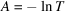,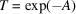]; vu is the volume of a primitive crystal unit cell; V is the volume of the crystal exposed to the beam; |F(hkl)|2 is the square of the structure-factor amplitude for the given reflection hkl; DA is the absorption of X-rays by the detector's active material; and DC is the detector's response to a single absorbed X-ray photon.

SCALEPACK determines the components of the total scale factor, i.e. the product of all factors that multiply the structure-factor amplitudes squared in equation (11.4.8.1):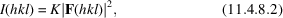where I is the intensity and K is the total scale factor.

All components of the total scale factor K can be calculated from non-diffraction measurements and calibration of the data-collection system. However, the absolute calibration of the whole system is rarely available and part of the scale factor (the overall scale factor ko) is determined by comparing the scaled data to the squared structure-factor amplitudes predicted from an atomic model obtained after the structure is solved: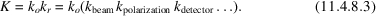The relative scale factor kr is calculated, but in practice we assume that some of its components are known from detector calibration, beam monitoring and the diffraction geometry. The scaling procedure determines the remaining parts of the scale factor (often iteratively), based on knowledge from subsequent stages of crystallographic analysis. When iterative procedures are applied, the scaling model has to include information about experimental uncertainties (discussed in Section 11.4.10).

SCALEPACK uses an exponential modelling approach (Otwinowski et al., 2003), which is flexible with regard to correlations among parameters and provides a uniform description of the parameter optimization process for various scaling models. In SCALEPACK scale factors si for each observation are calculated using a set of a priori unknown parameters pi: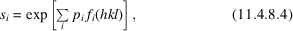where fi are pre-defined modelling functions of experimental conditions and i is a hierarchical index referring both to the type of correction and to the indices of the functional parameters describing this correction.

The simplest scaling model has a separate scale factor for each group (or batch) of data, e.g. one scale factor per image. In such a case,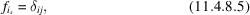where j is the batch index for a particular reflection. From equation (11.4.8.4)we obtain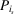as the logarithm of the scale factor of batch i.

To correct for a radiation-damage component represented as resolution-dependent decay, one temperature factor is used per batch of data,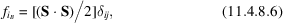where S is the scattering vector for each reflection. Using the same approach as for equation (11.4.8.5),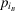is obtained as the relative temperature factor.

Another, more complex, multiplicative correction addresses unknown crystal absorption using an average of absorbances in the incoming and diffracted beam directions (Kopfmann & Huber, 1968). The correction is parameterized by real spherical harmonics as a function of the direction of the incoming beam and the diffraction vector S, expressed in the polar coordinate system of the rotating crystal (Katayama, 1986; Blessing, 1995):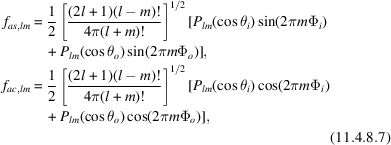where as, lm and ac, lm are parts of the hierarchical index i from equation (11.4.8.4); l, m are indices of the spherical harmonics; Plm is a Legendre polynomial; and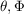are the polar coordinates of the incoming (index i) and the outgoing (index o) directions in the crystal coordinate system. The odd-order spherical harmonics should have zero coefficients when describing pure absorption of X-rays. However, due to correlation with other effects, the scaling may benefit from the inclusion of low-order odd-order harm­onics.

It is beneficial to correct even for a small discrepancy between the actual and assumed directions of the crystal rotation axis. The inaccuracy results in an error in the calculated value of the Lorentz factor [equation (11.4.8.1)]. The scale factor to correct for this error can be described using the parameter pl, the value of which represents a small angular error. The corresponding function is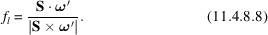There are additional effects that are parameterized using exponential modelling [equation (11.4.8.4)], for example, a correction for uneven crystal rotation and/or exposure (Otwinowski et al., 2003), or a correction for uneven detector response, which is very important for multi-CCD detectors. This approach can be extended to other experimental factors if there is a need to correct them during scaling without changing the overall logic of scale-factor determination (Otwinowski et al., 2003).

11.4.8.1. Global and local scaling

| top | pdf |

In principle, global scaling can be followed by local scaling (Matthews & Czerwinski, 1975). Local scaling is mostly applied to calculate differences of phasing signal, where it is assumed that a group of measurements, e.g. those close together in reciprocal space or detector space, should be on a similar scale. A flexible parameterization by the exponential modelling allows for a good description of all kinds of smooth corrections. Local scaling is much more limited in terms of what type of smooth variation is being corrected for, so it is unlikely to provide additional benefit to the general scaling method described here. In practice, if there is an improvement from such procedures at the stage of heavy-atom search, it implies that the scaling parameters for global scaling were not properly chosen.

11.4.8.2. Stabilization of scaling parameters based on prior knowledge

| top | pdf |

The unknown parameters in equation (11.4.8.4)are estimated with various level of uncertainty depending on the multiplicity of observations and how symmetry-equivalent reflections are related to each other. Potentially, this may result in unreasonable values of scaling parameters due to insufficient information to determine the values of parameters. In SCALEPACK, the method to stabilize such ill-conditioned calculations is closely related to Tikhonov stabilization (Tikhonov & Arsenin, 1977), where additional, a priori knowledge about the expected mag­nitude of the physical effect modelled is used to restrain the solutions, based on the same argument as in the case of restraints in the atomic refinement.

For example, logarithms of scale factors typically do not fluctuate by more than ws between frames, where expectation about ws is a function of the data-collection stability (beam stability, goniostat and/or crystal vibrations). This knowledge is described by adding a penalty term (scale restrain) to the functions being optimized: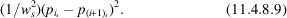A similar approach can be used in calculations of absorption coefficients. For smooth absorption with the expectation of decreasing magnitude of parameters for high orders of spherical harmonics [equation (11.4.8.7)], a reasonable restraint term parameterized by wa results in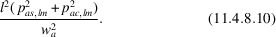If we do not want to penalize high-order terms more than the low-order ones, the following restraint can be used: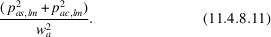References

Azaroff, L. V. (1955). Polarization correction for crystal-monochromatized X-radiation. Acta Cryst. 8, 701–704.
Blessing, R. H. (1995). An empirical correction for absorption anisotropy. Acta Cryst. A51, 33–38.
Katayama, C. (1986). An analytical function for absorption correction. Acta Cryst. A42, 19–23.
Kopfmann, G. & Huber, R. (1968). A method of absorption correction by X-ray intensity measurements. Acta Cryst. A24, 348–351.
Matthews, B. W. & Czerwinski, E. W. (1975). Local scaling: a method to reduce systematic errors in isomorphous replacement and anomalous scattering measurements. Acta Cryst. A31, 480–487.
Otwinowski, Z., Borek, D., Majewski, W. & Minor, W. (2003). Multi­parametric scaling of diffraction intensities. Acta Cryst. A59, 228–234.
Tikhonov, A. N. & Arsenin, V. I. A. (1977). Solutions of ill-posed problems. Washington, New York, Winston: Halsted Press.